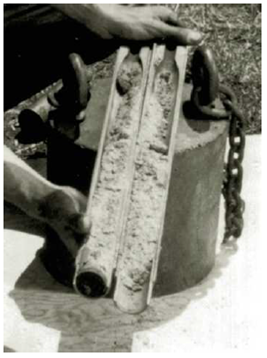Chapter 3, Problem 3.11PPrinciples of Geotechnical Enginee...

9th Edition
Braja M. Das + 1 other
ISBN: 9781305970939

Solutions

Chapter
SectionPrinciples of Geotechnical Enginee...

9th Edition
Braja M. Das + 1 other
ISBN: 9781305970939
Textbook Problem

During a subsurface exploration, an undisturbed soil sample was collected from the field using a split-spoon sampler for laboratory evaluation (see Figure 17.6 in Chapter 17). The sample has a diameter of 1.375 in., length of 18 in., and a moist weight of 1.85 lb. If the oven-dried weight was 1.5 lb and Gs = 2.74, calculate the following:a. Moist unit weightb. Moisture contentc. Dry unit weightd. Void ratioe. Degree of saturationFigure 17.6 Split-spoon sampler, unassembled (Courtesy of ELE International)

(a)

To determine

Calculate the moist unit weight of soil.

Explanation

Given information:

The diameter of the sample (d) is 1.375in..

The length of the sampler (L) is 18in..

The moist weight (W) of the sample is 1.85lb.

The oven-dried weight (WS) of the sample is 1.5lb.

The specific gravity of the soil solids (Gs) is 2.74.

Calculation:

Calculate the volume of the sampler (V) using the relation.

V=14πd2L

Substitute 1.375in. for d and 18in. for L.

V=14π×1

(b)

To determine

Calculate the moisture content.

(c)

To determine

Calculate the dry unit weight of soil.

(d)

To determine

Calculate the void ratio of soil.

(e)

To determine

Calculate the degree of saturation.

Still sussing out bartleby?

Check out a sample textbook solution.

See a sample solution

The Solution to Your Study Problems

Bartleby provides explanations to thousands of textbook problems written by our experts, many with advanced degrees!

Get Started

List three safety precautions to be observed when using a hacksaw.

Precision Machining Technology (MindTap Course List)

What is sparse data? Give an example.

Database Systems: Design, Implementation, & Management

What is natural language processing?

Fundamentals of Information Systems

Given that P=120lb and Q=130lb, find the rectangular representation of P+Q.

International Edition---engineering Mechanics: Statics, 4th Edition

What is a common problem in calculating start and finish times? Provide an example.

Systems Analysis and Design (Shelly Cashman Series) (MindTap Course List)

Why is the oxygen valve turned on before starting to clean a cutting tip?

Welding: Principles and Applications (MindTap Course List)

List uses of GPS.

Enhanced Discovering Computers 2017 (Shelly Cashman Series) (MindTap Course List)